Circles Part 1

### Circle Generators

Many incremental methods exist to plot circles and arcs.
1) If the hardware exists for line generation, then circles are generated using short line segments.
2) If the line generation hardware does not exist, then circles are generated using closely spaced dots.

#### Central Symmetry

A usable fact is that if the center of the circle is at location (0,0) and a point (x,y) lies on the circumference of the circle, then we know 7 more points on the circle.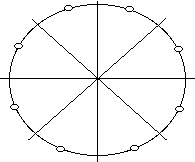We can set eight symmetric pixels that correspond to a point (x,y) on the circle using the following procedure:

procedure set_eight(x,y,xcenter,ycenter:integer);
begin
plot_pixel(x+xcenter,y+ycenter);
plot_pixel(-x+xcenter,y+ycenter);
plot_pixel(x+xcenter,-y+ycenter);
plot_pixel(-x+xcenter,-y+ycenter);
plot_pixel(y+xcenter,x+ycenter);
plot_pixel(-y+xcenter,x+ycenter);
plot_pixel(y+xcenter,-x+ycenter);
plot_pixel(-y+xcenter,-x+ycenter);
end;

The book discusses the pixel ratio problem. Essentially, the pixel ratio can be defined as the distance between the centers of two horizontal pixels divided by the distance between the centers of two vertical pixels. In the past, the distance between the horizontal adjacent pixels has been less than the vertical. Presently, the pixel ratio of most monitors is 1 and so you can skip the section "Correction for pixel ratio".

### Circle Generating Algorithms

Non-DDA Methods

1) Parameter Method - this method consists of calculating values (x,y) on the circle using the following formulas:

x = rcos(u)
y = rsin(u)

where u increases incrementally by small steps over the interval 0 to 2(pi). We can use symmetry to improve the execution time so that u is calculated over the interval 0 to pi/4. We also note that if the incremental value of u is <= 1/r, where r is the length of r expressed in pixels, the circle generator advances by approximately one pixel each iteration. This algorithm is slow because of the constant recalulation of sin(u) and cos(u).

As an example, let's assume that we are generating a circle from the origin with radius 10. This would imply the following table of values:

```u	X=rcos(u)	Y=rsin(u)
0	10		0
.1	9.95		.999
.2	9.8		1.99
.3	9.55		2.96
.4	9.21		3.89
.5	8.78		4.79
.6	8.25		5.65
.7	7.65		6.44
.8	6.97		7.17  (Note: pi/4 = .7854)
....
1.5708	0		10    (Note: pi/2 = 1.5708)
```
2) Rotation Method - this method consists of using the point (x,y) on the circle and rotating it about the origin. The following equations will produce the desired result:

a) Clockwise

Xrot = Xcos(u) + Ysin(u)
Yrot = -Xsin(u) + Ycos(u)

b) Counterclockwise

Xrot = Xcos(u) - Ysin(u)
Yrot = Xsin(u) + Ycos(u)

Note: The book is in error!!

To use the rotation method, we assign (X0,Y0) equal to (r,0). We can then use the following equations to generate successive points:

Xn+1 = Xncos(u) - Ynsin(u)
Yn+1 = Xnsin(u) + Yncos(u)

Discussion Questions:
1) Compare and contrast the Parameter Method and the Rotation Method. Which method would you use and why?
2) What is the terminating condition for the Rotation Method?
3)Can you think of a way to speed the Rotation Method up?

DDA Method

Bresenham's Circle Generation

This circle generation algorithm uses only integer arithmetic and is based on the differential equation of a circle. I will skip this explanation for now. The algorithm as we will discuss it, generates the portion of the circle from 90 degrees to 45 degrees. The circle is at the origin with radius r and generates one new pixel per iteration.

Consider an arbitrary point on the circle (Xn,Yn). The next point on the circle is either (Xn + 1,Yn) or (Xn + 1,Yn -1). Pictorially, this looks like: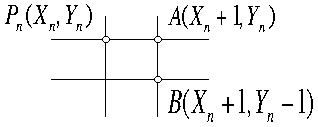How is the next pixel chosen? In general, we know that the equation of a circle is:
(X-h)^2 + (Y-k)^2 = r^2

What can you deduce from this general form?

We can somewhat restrict the general equation of a circle to the equation about the origin as follows:
X^2 + Y^2 = r^2

It turns out that we will select the next pixel based on a test using the function:

f(X,Y) = X^2 + Y^2 - r^2 as follows:

1) all points (x,y) that satisfy f(x,y)=0 lie exactly on the circle.
2) all points (x,y) that satisfy f(x,y)>0 lie outside the circle.
3) all points (x,y) that satisfy f(x,y)<0 lie inside the circle.

Five possibilities exist with the points Pn, A, and B as follows: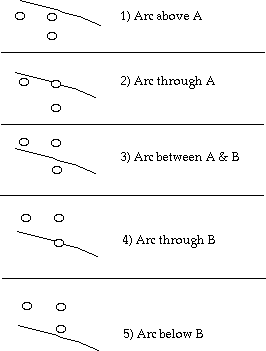The fact is that we plotted the point Pn and we are looking to plot the next point. The next point will be either A or B and we must use the available information to make that decision. We remember that the point A is (Xn + 1, Yn) and the point B is (Xn + 1, Yn -1), so looking at the sum = f(A) + f(B), we can determine the next point that we wish to plot.

```	1	2	3	4	5

f(A)	neg	zero	pos	pos	pos
f(B)	neg	neg	neg	zero	pos
sum	neg	neg	?	pos	pos

```

This implies that to make the correct choice, we need to look at the sign of the sum of the two functions, f(A) & f(B).

Consider the following: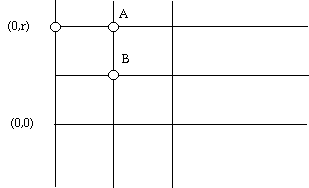If the starting pixel is P0=(X0,Y0)=(0,r), we need to look at f(A)+f(B) to make a decision about whether to choose A or B. So what is f(A)+f(B) in general if P0=(0,r)?

Remember, the following equations determine how pixels A and B lie with respect to the circle

f(A)=(Xn + 1)^2 + Yn^2 - r^2
f(B)=(Xn + 1)^2 + (Yn - 1)^2 - r^2

Problem: Given a circle whose center is (0,0) with a radius of 10, determine the next point on the circle to be plotted and explain why.

Once we have determined which point A or B is to be plotted, we need to calculate the next sum (f(A)+f(B)) from that point. In particular, we would like to find the next sum needed to go from P1 to P2 such that it can be expressed in terms of the old sum. In general, we would like to show the case Pn with coordinates (Xn,Yn) and consider how the next two pixels are chosen. If this seems confusing, it is. Before deriving this, let's take a look at Bresenham's Circle Generating Algorithm and work through some steps.

```procedure brescircle(radius,xcenter,ycenter:integer);
var xstart,ystart,sum:integer;
begin
xstart := 0;
while(xstart <= ystart) do
begin
set_eight(xstart,ystart,xcenter,ycenter);
if(sum <= 0) then
sum := sum + 4*xstart + 6;
else
begin
sum := sum + 4*(xstart-ystart) + 10;
ystart := ystart - 1;
end;<
xstart := xstart + 1;
end;
end;
```
Problem: Show the first four points generated from the starting point (0,10) using Bresenham's algorithm.

Question: Where do the following two statements come from:
1) sum := sum + 4*xstart + 6
2) sum := sum + 4*(xstart-ystart) + 10

Consider the following diagram: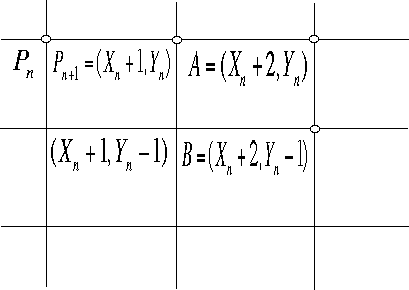Pn is the point just plotted. From the picture we can see that if the sum < 0, then we continue on with pixel Pn+1. Notice that the coordinates for Pn+1 are (Xn +1,Yn). The case of sum=0 and sum>0 will be left for your enjoyment.

The expression for s from a point (Xn,Yn) to point A or B is:

```sum =  (Xn + 1)^2 + Yn^2 - r^2 +      {this is f(A)}
(Xn + 1)^2 + (Yn - 1)^2 - r^2  {this is f(B)}

sum = 2Xn^2 + 2Yn^2 + 4Xn - 2Yn - 2r^2 + 3
```

If (sum < 0) then we continue with Pn+1 = (Xn + 1,Yn)
else we continue with Pn+1 = (Xn + 1,Yn - 1)

Since we are assuming that the sum is less than zero, we continue on with the point (Xn + 1,Yn).

In order to go from here to Pn+2 which can be A or B, once again the value of the sum is computed as follows:

```sum_new = (Xn + 2)^2 + Yn^2 - r^2 +       {f(A)}
(Xn + 2)^2 + (Yn - 1)^2 - r^2   {f(B)}

sum_new = 2Xn^2 + 2Yn^2 + 8Xn - 2Yn - 2r^2 +9

```
What's interesting is that a closer look at sum_new shows that it can be written in terms of sum. That is:

sum_new = sum + 4Xn + 6

We can similarly define sum_new for the case when sum >= 0 as:

sum_new = sum + 4*(Xn - Yn) + 10

What is the expense of both of these operations?